# Engineering Mechanics - Equilibrium of a Particle

## Why Engineering Mechanics Equilibrium of a Particle?

In this section you can learn and practice Engineering Mechanics Questions based on "Equilibrium of a Particle" and improve your skills in order to face the interview, competitive examination and various entrance test (CAT, GATE, GRE, MAT, Bank Exam, Railway Exam etc.) with full confidence.

## Where can I get Engineering Mechanics Equilibrium of a Particle questions and answers with explanation?

IndiaBIX provides you lots of fully solved Engineering Mechanics (Equilibrium of a Particle) questions and answers with Explanation. Solved examples with detailed answer description, explanation are given and it would be easy to understand. All students, freshers can download Engineering Mechanics Equilibrium of a Particle quiz questions with answers as PDF files and eBooks.

## Where can I get Engineering Mechanics Equilibrium of a Particle Interview Questions and Answers (objective type, multiple choice)?

Here you can find objective type Engineering Mechanics Equilibrium of a Particle questions and answers for interview and entrance examination. Multiple choice and true or false type questions are also provided.

## How to solve Engineering Mechanics Equilibrium of a Particle problems?

You can easily solve all kind of Engineering Mechanics questions based on Equilibrium of a Particle by practicing the objective type exercises given below, also get shortcut methods to solve Engineering Mechanics Equilibrium of a Particle problems.

### Exercise :: Equilibrium of a Particle - General Questions

• Equilibrium of a Particle - General Questions
1.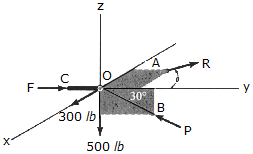The joint O of a space frame is subjected to four forces. Strut OA lies in the x-y plane and strut OB lies in the y-z plane. Determine the force acting in each if the three struts required for equilibrium of the joint. Set= 45°.

 A. F = 46.4 lb, R = 400 lb, P = 424 lb B. F = 566 lb, R = 424 lb, P = 1000 lb C. F = 11.3 lb, R = 424 lb, P = 577 lb D. F = 1166 lb, R = 424 lb, P = 1000 lb

Explanation:

No answer description available for this question. Let us discuss.

2.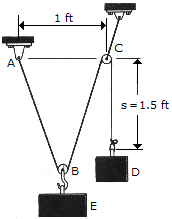A "scale" is constructed with a 4-ft-long cord and the 10-lb block D. The cord is fixed to a pin at A and passes over two small pulleys at B and C. Determine the weight of the suspended block E if the system is in equilibrium when s = 1.5 ft.

 A. W = 8.01 lb B. W = 14.91 lb C. W = 17.63 lb D. W = 18.33 lb

Explanation:

No answer description available for this question. Let us discuss.

3.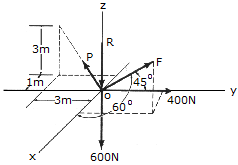Determine the magnitudes ofthe forces P, R, and F required for equillibrium of point O.

 A. R = 238 N, F = 181.0 N, P = 395 N B. R = 1340 N, F = 740 N, P = 538 N C. R = 419 N, F = 181.0 N, P = 395 N D. R = 409 N, F = 504 N, P = 1099 N

Explanation:

No answer description available for this question. Let us discuss.

4.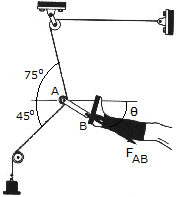Determine the magnitude and directionof the resultant force FAB exerted along link AB by the tractive apparatus shown. The suspended mass is 10 kg. Neglect the size of the pulley at A.

 A. FAB = 170.0 N,= 75.0° B. FAB = 170.0 N,= 15.0° C. FAB = 98.1 N,= 75.0° D. FAB = 98.1 N,= 15.0°

Explanation:

No answer description available for this question. Let us discuss.

5.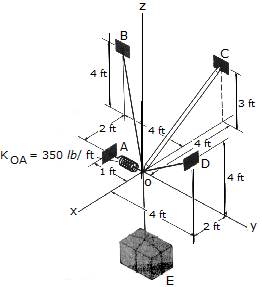Determine the tension developed in cables OD and OB and the strut OC, required to support the 500-lb crate. The spring OA has an unstretched length of 0.2 ft and a stiffness of kOA = 350lb/ft. The force in the strut acts along the axis of the strut.

 A. Fob = 289 lb, Foc = 175.0 lb, Fod = 131.3 lb B. Fob = 86.2 lb, Foc = 175.0 lb, Fod = 506 lb C. Fob = 375 lb, Foc = 0, Fod = 375 lb D. Fob = 664 lb, Foc = 175.0 lb, Fod = 244 lb